# Chapter 1 : Mechanical Engineering and Mechanics

### Topics covered in this snack-sized chapter:

#### What is Mechanical Engineering arrow_upward

• “Mechanical engineering is the branch of engineering that deals with forces and motion.”
• It includes designing, analyzing, developing, and maintaining mechanical systems.
• Mechanical engineers apply the principles of mechanics, materials and energy to design machines and devices.

• #### Sub Categories arrow_upward

• Mechanics
• Thermodynamics
• Mechatronics
• Structural analysis
• Mechanical Design

• ###### Mechanics

• The study of forces acting on physical bodies is known as Mechanics.

• ###### Thermodynamics

• Thermodynamics is the study of transformations of energy.

• ###### Mechatronics

• Mechatronics is a design process that includes a combination of mechanical engineering, electrical engineering, control engineering and computer engineering.

• #### Core Concepts arrow_upward

• Mechanical Engineering field requires an understanding of core concepts including:
• Kinematics
• Dynamics
• Strength of materials
• Thermodynamics
• Automotive engines
• Fluid mechanics and fluid dynamics
• Mechanism design
• Manufacturing engineering, technology, or processes
• Hydraulics and pneumatics
• Engineering design
• Product design
• Mechatronics and control theory
• Material Engineering
• Computer-aided manufacturing (CAM)

#### History and Time Line arrow_upward

• Mechanical Engineering is one of the oldest Engineering branch just behind Civil Engineering.
• The development of the steam engine in late 17th century provided power to fuel the Industrial Revolution.
• James Watt developed a steam piston engine in 1775.
• Stirling engine was developed in 1816.
• It uses temperature difference to produce mechanical work.
• The Otto cycle engine was developed in 1876 by Nikolaus August .This engine has four stroke cycles.
• Diesel Engine was invented by Rudolf Diesel in 1893.
• The Diesel engine has the lowest specific fuel consumption of any large internal combustion engine.

#### Classification of Mechanics arrow_upward

• Depending upon the nature of the body involved, it can be further divided into-
• Mechanics of solids
• Mechanics of fluids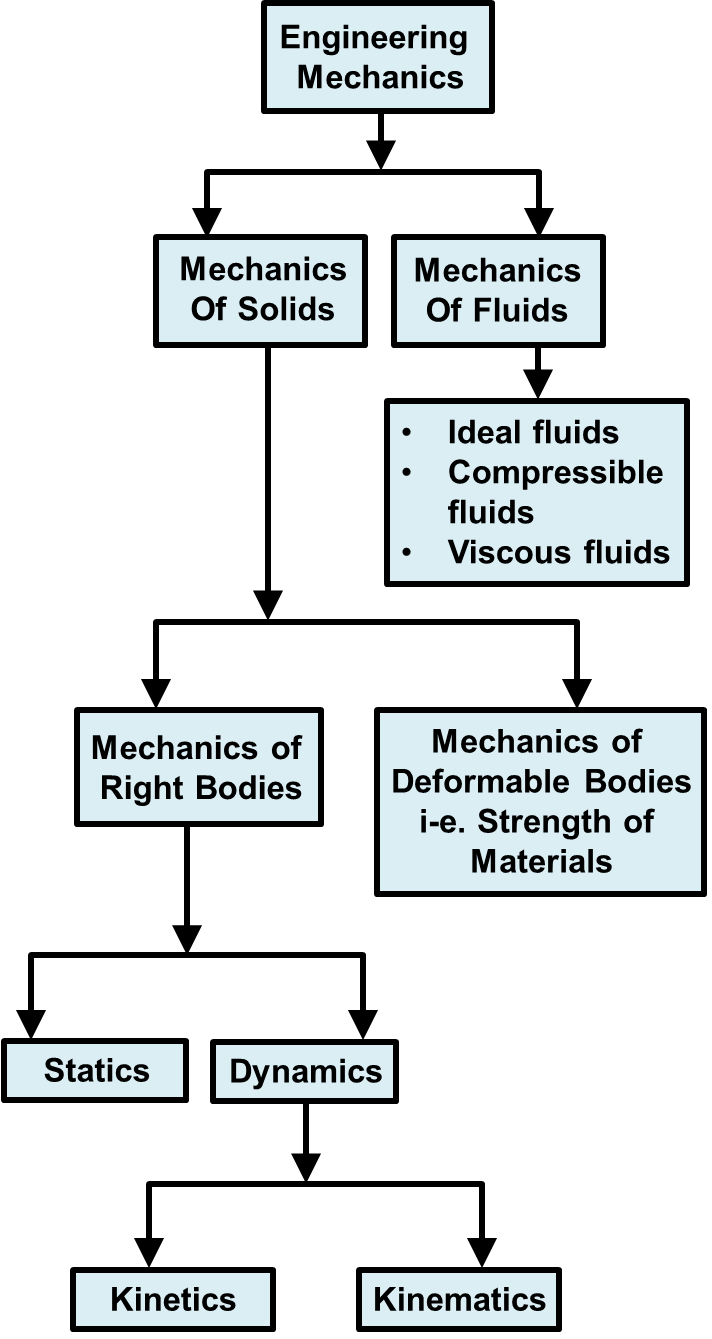#### Mechanics of Solids arrow_upward

• The Mechanics of Solids or Rigid Bodies is sub-divided into two branches:
• Statics
• Dynamics
##### Statics
• Statics is the branch of mechanics under which we study the effect of forces on rigid bodies which are at rest and remain at rest or have uniform motion.
•  Such bodies are said to be in equilibrium.
• ##### Dynamics
• Dynamics is the branch of mechanics under which we study the effect of forces and motion of rigid bodies.
• It may be classified as following:
• Kinetics - It is the study of relationship between the forces and the resulting motion.
• Kinematics - It is the study of motion of the body without any reference to the force causing motion or forces produced as a result of the motion.

#### Young’s Modulus arrow_upward

• Young's Modulus is the ratio of tensile stress to tensile strain.
• Young's modulus has units of pressure.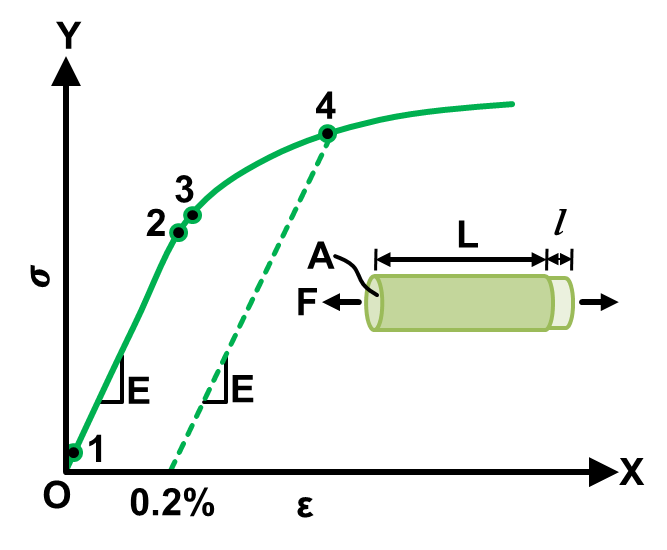• The numbers in the above graph represents following:
• 1- True elastic limit
• 2- Proportionality limit
• 3- Elastic limit
• 4- Offset yield strength

###### Calculation of Young’s Modulus:

• Young's modulus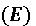can be calculated by dividing the tensile stress by the tensile strain in the elastic (initial, linear) portion of the stress-strain curve:
•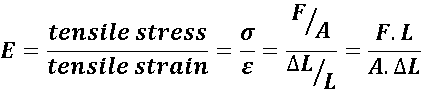Where,

• E is the Young's modulus.
• F is the force exerted on the object under tension.
• A is the original cross-sectional area through which the force is applied.
•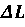is the amount by which the length of the object changes (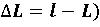.
• L is the original length of the object.

#### Mechanics of Fluids arrow_upward

• It is the study of liquids and gases i.e. fluids at rest or in motion.
• Fluids can be classified as compressible or incompressible.
• If the density of the fluid varies with temperature & pressure, fluid is said to be compressible.

• #### Free-Body Diagrams arrow_upward

• A free body diagram, also called a force diagram, is a pictorial representation often used by physicists and engineers to analyze the forces acting on a body of interest.
• A free body diagram to show all the forces acting on a body is given below:
•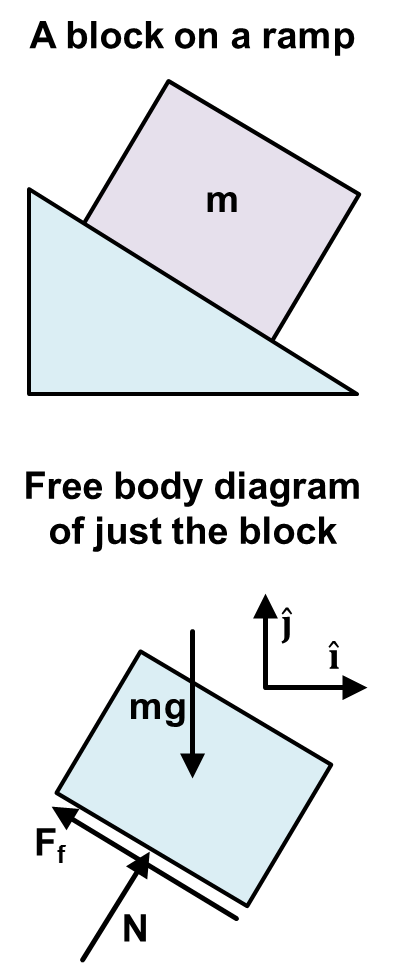#### Trusses arrow_upward

• A Truss is a structure comprising one or more triangular units constructed with straight members whose ends are connected at joints referred to as nodes.
• External forces and reactions to those forces are considered to act only at the nodes and result in forces in the members which are either tensile or compressive forces.
• Bridges, roof supports, derricks, and other such structures are common example of trusses.

• ###### Types of Trusses

• Plane Truss:
• A Plane Truss is one where all the members and nodes lie within a two dimensional plane or single plane.
• Basic element of a plane truss is triangle.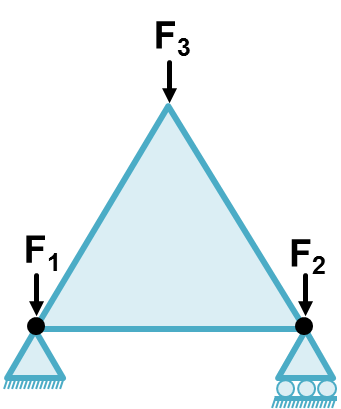• The commonly used examples of plane trusses are Pratt, Howe, Warren, Baltimore, and Fink Trusses etc.
• Space Truss:
• A Space Frame Truss is a three-dimensional framework of members pinned at their ends.
• A tetrahedron shape is the simplest space truss, consisting of six members which meet at four joints.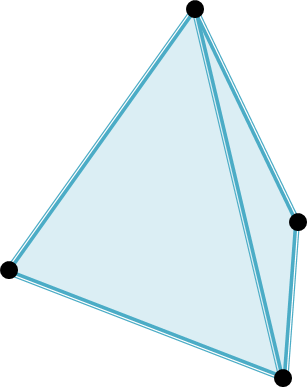Space frame truss

#### Virtual Work arrow_upward

• Total work done by the external forces when virtual displacements are made in ‘n’ particle system, is known as virtual work.
• If a particle, rigid body, or a system of rigid bodies which is in equilibrium under various forces is given an arbitrary virtual displacement, the net work done by the external forces during that displacement is zero.
• If Fi a be the applied force and fi be the constraint force acting on i th particle, the net force acting on the system is
•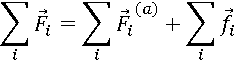• If dri is the virtual displacement, the work done on the system is: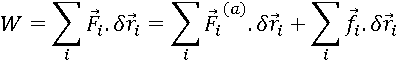When system is in equilibrium,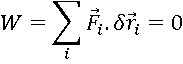Also,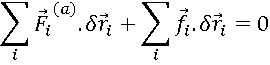• The virtual displacements dri are such that the constraint forces do no work ().

Thus,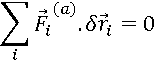• The condition for static equilibrium is that the virtual work done by all the applied forces should vanish, provided the virtual work done by all the constraint forces vanishes. This is called the principle of virtual work.

• #### Importance of Mechanics arrow_upward

The research in applied Mechanics has wide applications in many departments of engineering like:

• Civil
• Mechanical
• Construction
• Material Science and engineering
• Aerospace
• Chemical
• Electrical
• Nuclear
• Structure and Bioengineering

• #### Thank You from Kimavi arrow_upward

• Please email us at Admin@Kimavi.com and help us improve this tutorial.